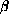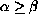Electron. J. Diff. Eqns., Vol. 2005(2005), No. 03, pp. 1-10.

### Existence and approximation of solutions of second order nonlinear Neumann problems Rahmat Ali Khan

Abstract:
We study existence and approximation of solutions of some Neumann boundary-value problems in the presence of an upper solutionand a lower solutionin the reversed order (). We use the method of quasilinearization for the existence and approximation of solutions. We also discuss quadratic convergence of the sequence of approximants.

Submitted November 6, 2004. Published January 2, 2005.
Math Subject Classifications: 34A45, 34B15.
Key Words: Neumann problems; quasilinearization; quadratic convergence.

Show me the PDF file (193K), TEX file, and other files for this article.

 Rahmat Ali Khan Department of Mathematics, University of Glasgow Glasgow G12 8QW, UK email: rak@maths.gla.ac.uk